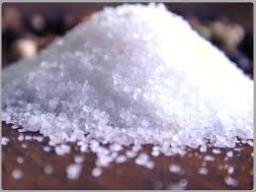# Kilograms 3094

1.5 kg of a 20% NaCl solution should be diluted with water to a 10% solution. How much water will we need, and how many kilograms of diluted solution will we get?

x =  1.5
y =  3

### Step-by-step explanation:

1.5·0.20 + 0·x = 0.10·y
y = 1.5+x

0.1y = 0.3
x-y = -1.5

Pivot: Row 1 ↔ Row 2
x-y = -1.5
0.1y = 0.3

y = 0.3/0.1 = 3
x = -1.5+y = -1.5+3 = 1.5

x = 3/2 = 1.5
y = 3

Our linear equations calculator calculates it.Did you find an error or inaccuracy? Feel free to write us. Thank you!

Tips for related online calculators